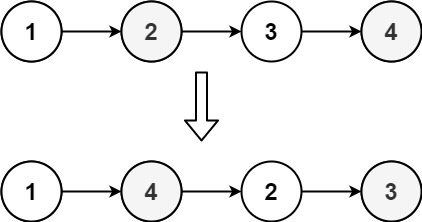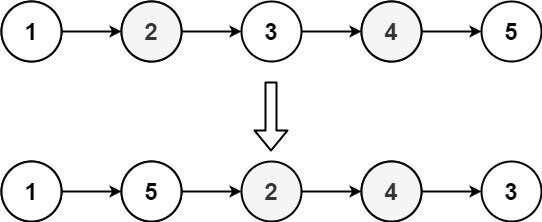304 North Cardinal St.
Dorchester Center, MA 02124

# Reorder List LeetCode Solution

## Problem – Reorder List LeetCode Solution

You are given the head of a singly linked-list. The list can be represented as:

``````L0 → L1 → … → Ln - 1 → Ln
``````

Reorder the list to be on the following form:

``````L0 → Ln → L1 → Ln - 1 → L2 → Ln - 2 → …
``````

You may not modify the values in the list’s nodes. Only nodes themselves may be changed.

Example 1:``````Input: head = [1,2,3,4]
Output: [1,4,2,3]
``````

Example 2:``````Input: head = [1,2,3,4,5]
Output: [1,5,2,4,3]
``````

Constraints:

• The number of nodes in the list is in the range `[1, 5 * 104]`.
• `1 <= Node.val <= 1000`

## Reorder List LeetCode Solution in Java

``````public void reorderList(ListNode head) {

//Find the middle of the list
while(p2.next!=null&&p2.next.next!=null){
p1=p1.next;
p2=p2.next.next;
}

//Reverse the half after middle  1->2->3->4->5->6 to 1->2->3->6->5->4
ListNode preMiddle=p1;
ListNode preCurrent=p1.next;
while(preCurrent.next!=null){
ListNode current=preCurrent.next;
preCurrent.next=current.next;
current.next=preMiddle.next;
preMiddle.next=current;
}

//Start reorder one by one  1->2->3->6->5->4 to 1->6->2->5->3->4
p2=preMiddle.next;
while(p1!=preMiddle){
preMiddle.next=p2.next;
p2.next=p1.next;
p1.next=p2;
p1=p2.next;
p2=preMiddle.next;
}
}
``````

## Reorder List LeetCode Solution in C++

``````class Solution {
public:
void reorderList(ListNode* head) {
if ((!head) || (!head->next) || (!head->next->next)) return; // Edge cases

stack<ListNode*> my_stack;
ListNode* ptr = head;
int size = 0;
while (ptr != NULL) // Put all nodes in stack
{
my_stack.push(ptr);
size++;
ptr = ptr->next;
}

ListNode* pptr = head;
for (int j=0; j<size/2; j++) // Between every two nodes insert the one in the top of the stack
{
ListNode *element = my_stack.top();
my_stack.pop();
element->next = pptr->next;
pptr->next = element;
pptr = pptr->next->next;
}
pptr->next = NULL;
}
};
``````

## Reorder List LeetCode Solution in Python

``````class Solution:
#step 1: find middle
if not head: return []
while fast.next and fast.next.next:
slow = slow.next
fast = fast.next.next

#step 2: reverse second half
prev, curr = None, slow.next
while curr:
nextt = curr.next
curr.next = prev
prev = curr
curr = nextt
slow.next = None

#step 3: merge lists
``````
##### Reorder List LeetCode Solution Review:

In our experience, we suggest you solve this Reorder List LeetCode Solution and gain some new skills from Professionals completely free and we assure you will be worth it.

If you are stuck anywhere between any coding problem, just visit Queslers to get the Reorder List LeetCode Solution

Find on Leetcode

##### Conclusion:

I hope this Reorder List LeetCode Solution would be useful for you to learn something new from this problem. If it helped you then don’t forget to bookmark our site for more Coding Solutions.

This Problem is intended for audiences of all experiences who are interested in learning about Data Science in a business context; there are no prerequisites.

Keep Learning!

More Coding Solutions >>

LeetCode Solutions

Hacker Rank Solutions

CodeChef Solutions